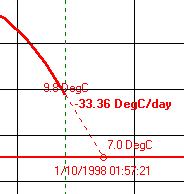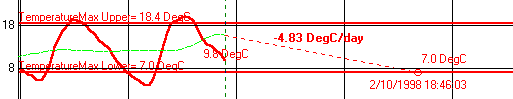# PredictionsThe date and time when the sensor will reach the lower limit (if it is increasing) or the upper limit (if it is increasing) can be calculated and plotted.

The rate of change that will be used to calculate the predicted date can be from...

• The last 2 readings
• The rate calculation
• The smooth calculation

The user can also enter their estimate of the rate, and M-Log will calculate a date based on this rate.If last 2 is chosen the calculations will be made from the latest smoothed value.

Use to set options, and see Scheduling for more options.